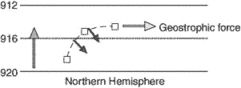# geostrophic wind speed

## geostrophic wind speedWinds exist because of horizontal and vertical pressure gradient so atmospheric motion can be deduced from isobaric surface charts etc. If the horizontal pressure gradient force is exactly balanced in magnitude by Coriolis effect accelerations of the air will be relatively small and a geostrophic wind will flow horizontally at a constant speed proportional to the isobaric spacing gradient, perpendicular to the two opposing forces and parallel to straight isobars. Thus geostrophic wind speed is proportional to the pressure gradient. The closer the isobars, the higher will be geostrophic wind speed.
The speed of a geostrophic wind calculated from the pressure gradient, air density, rotational velocity of the earth, and latitude. The calculation ignores the curvature of the wind's path. A geostrophic wind is proportional to the pressure gradient or inversely proportional to the distance between the isobars.
Mentioned in ?
References in periodicals archive ?
20CR, on the other hand, seems to underestimate the wind speeds at 800 hPa: a rough calculation of the geostrophic wind speed in the region of the two stations with the strongest winds in Fig.
The most popular way consists in the use of geostrophic wind fields that are adjusted to the 10 m level by means of a simplified procedure in which the geostrophic wind speed (usually retrieved from the Swedish Meteorological and Hydrological Institute database) was multiplied by 0.
The wind forcing at a 10 m level was derived from geostrophic winds as recommended by Bumke and Hasse (1989): the geostrophic wind speed was multiplied by 0.
An approximation of the near-surface wind at the 10 m level, used as the input to the wave model, was calculated following a standard procedure in which the geostrophic wind speed was multiplied by 0.

Site: Follow: Share:
Open / Close